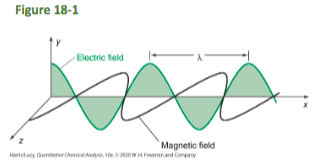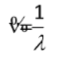# Quantitative Chemical Analysis: CH 18 Fundamentals of Spectrophotometry Flashcards

Set Details Share
created 3 weeks ago by c_mylinh
5 views
Chapter Outline • Section 181 Properties of Light • Section 182 Absorption of Light • Section 183 Measuring Absorbance • Section 184 Beer's Law in Chemical Analysis • Section 185 Spectrophotometric Titrations • Section 186 What Happens When a Molecule Absorbs Light?
updated 3 weeks ago by c_mylinh
Subjects:
science, chemistry, analytic
Page to share:
Embed this setcancel
COPY
code changes based on your size selection
Size:
X

1

Spectroscopy

the theoretical approach to the science of studying the interaction between matter and radiated energy

2

Spectrometry

the practical application of spectroscopy

3

Spectrometry uses instruments called

spectrometers

4

Spectrophotometry

the method used to measure how much achemical substance absorbs light as a beam of light passes through asample solution

5

Spectrophotometers

the instruments used to quantitatively measure the reflection or transmission properties of a material as a function of wavelength (a spectrum)

6

Spectrum

the reflection or transmission properties of a material as a function of wavelength

7

Light behaves as: (2)

a WAVE and a PARTICLE

8

Light as a WAVE

- has a wavelength and frequency

- exhibits the wave phenomena of interference, diffraction, and reflection.

- Wave properties govern light behavior such as interference and diffraction

9

Wave properties govern light behavior such as

interference and diffraction

10

Light as a PARTICLE (a photon)

- carries a discrete energy that can be absorbed or emitted by a molecule.

- The interaction of light with chemicals is described using the particle nature of light—the photon and its energy

11

Light waves consist ofperpendicular, oscillating electric and magnetic fields

12

Wavelength, λ

the distance between wave crests

13

Wavelength units

m, µm, nm

14

Wavenumber (v)a measure of spatial frequency

(v = 1/λ)

15

Frequency, ν

• the number of oscillations per second of the wave
• Frequency has
units of hertz (s-1).
• 1 Hz = 1 oscillation per second

16

Speed of light, c, formula

• The product of wavelength times frequency
• νλ = c
• In a vacuum, all light travels at the same speed.
c = 2.998 × 108 m/s

17

Light has a duality of

Wave-Particle

18

What is the smallest amount of light that can be generated by a light source?

A photon is the smallest amount of light that can be generated by a light source.
A photon is a particle of light.

19

The energy of a photon can be calculated from its

frequency

20

Photon energy formula

Ephoton = hν

where

h = Planck’s constant = 6.626 × 10-34 J∙s
ν = the frequency of the photon in units of hertz, s-1
E = the energy of the photon in units of joules, J

21

Constants

c = 2.998 × 108 m/s (the speed of light in a vacuum)
h = 6.626 × 10-34 J∙s (Planck’s constant)

22

Equations

v = 1/λ (conversion equation between wavelength and wavenumbers)

λν = c (equation for speed of light in a vacuum)

Ephoton = hν (equation for energy of a photon of light)

E = hν = hc/λ = hcv

23

Our eyes only see a fraction of the light in the universe. T/F?

True

24

Spectrophotometry

Any technique that uses light to measure chemical concentrations

25

Absorption Spectrophotometry

Any technique that uses the absorption of light to measure chemical
concentrations